13.1 Creating a node

Problem

I wish to create a node in HARK by myself but do not understand how to do so using only the material from a HARK training session.

Solution

To create a new node, it is necessary to install HARK by compiling a source, not by a debian package. To install from a source compilation, see “Installation of HARK” in the HARK training session material. When it is ready, describe the source of the node to be created. To learn how to make a basic node, consult the following items included in “Creation of a node” in the HARK training session material:

• Basic form of cc file (source file)

• Description with examples (ChannelSelector )

• Rewriting method of Makefile.am

This section further describes the following items showing the actual creation of nodes such as PublisherInt.cc and SubscriberInt.cc

• Buffer (Lookback Lookforward)

• Input-output of each type

• Switching the number of inputs from static to configurable

Creation of PublisherInt.cc

First, create PublisherInt.cc, which reads integers as a parameter and discharges them without change. (note: the hark_test directory is assumed as a package.) Cut and paste the following source code to

{$PACKAGE}/hark_test/src/PublisherInt.cc. #include <iostream> #include <BufferedNode.h> #include <Buffer.h> using namespace std; using namespace HARK; class PublisherInt; DECLARE_NODE(PublisherInt); /*Node * * @name PublisherInt * @category HARK_TEST * @description This block outputs the same integer as PARAM1. * * @output_name OUTPUT1 * @output_type int * @output_description This output the same integer as PARAM1. * * @parameter_name PARAM1 * @parameter_type int * @parameter_value 123 * @parameter_description Setting for OUTPUT1 * END*/ class PublisherInt : public BufferedNode { int output1ID; int output1; int param1; public: PublisherInt(string nodeName, ParameterSet params) : BufferedNode(nodeName, params) { output1ID = addOutput("OUTPUT1"); output1 = 0; param1 = dereference_cast<int>(parameters.get("PARAM1")); inOrder = true; } void calculate(int output_id, int count, Buffer &out) { // Main loop routine starts here. output1 = param1; cout << "Published : [" << count << " , " << output1 << "]" << endl; (*(outputs[output1ID].buffer))[count] = ObjectRef(Int::alloc(output1)); // Main loop routine ends here. } }; Each part of the source code is described below. #include <iostream> #include <BufferedNode.h> #include <Buffer.h> Make sure to include a library of standard output and a library for HARK when creating a node. using namespace std; using namespace HARK; Declaration of a name space. Since all the classes of HARK , the basis of HARK, are defined in the name spaces of HARK, make sure to declare them when abbreviating. class PublisherInt; A class name of this node must be the same as the node name set in the following. DECLARE_NODE(PublisherInt); /*Node * * @name PublisherInt * @category HARK_TEST * @description This block outputs the same integer as PARAM1. * * @output_name OUTPUT1 * @output_type int * @output_description This output the same integer as PARAM1. * * @parameter_name PARAM1 * @parameter_type int * @parameter_value 123 * @parameter_description Setting for OUTPUT1 * END*/ In DECLARE_NODE, make sure that the PublisherInt class is defined as one node (an error will occur if it is not the same as the class name). @name seen in the comment out below is the setting for the declared node on GUI of HARK-Designer. It is not a comment, so make sure to set it. Four values are must be set: 1) the main body of the node, 2) the node inputs, 3) the node outputs, and 4) the node internal parameters. Other than the setting of 1), multiple values can be used (the setting method for multiple values are described later). The following are the concrete set values. • Setting of the main body of the node • @name: Node name indicated on HARK (should be the same as the class name) • @category: Setting of the category to which the node belongs on GUI of HARK-Designer. • @description: Description of the node (can be omitted) • Setting of node inputs • @input_name: Name of input indicated in the node • @input_type: Type of input variable • @input_description: Description of the input variable (can be omitted) • Setting of node outputs • @output_name: Name of output indicated in the node • @output_type: Type of output variable • @output_description: Description of the output variable (can be omitted) • Setting of internal parameter of the node • @parameter_name: Name of the parameter indicated in the node (indicated in a window when placing the mouse over it) • @parameter_type: Type of parameter • @parameter_value: Initial value of the parameter (can be changed in the source) • @parameter_description: Description of the parameter (can be omitted). This source has one output and one internal parameter, and therefore they are displayed as Fig. 13.1 in HARK-Designer.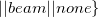Figure 13.1: PublisherInt node class PublisherInt : public BufferedNode { int output1ID; int output1; int param1; Define the PublisherInt class that inherits the BufferedNode class, with the latter defined in HARK . outputID is an integer that stores an ID of an output port. The pointer to be passed to the output port is obtained based on this ID. public: PublisherInt(string nodeName, ParameterSet params) : BufferedNode(nodeName, params) { output1ID = addOutput("OUTPUT1"); output1 = 0; param1 = dereference_cast<int>(parameters.get("PARAM1")); inOrder = true; } The constructer that inherits the BufferedNode class. nodeName (the class object name in the network files of HARK ) and params (an initializer of the variable parameters contained within the Node class and with internal parameters defined for some) are used as arguments. output1ID = addOutput("OUTPUT1"); becomes a row that stores the ID of OUTPUT1 set in the HARK-Designer GUI in output1ID defined in the class. param1 = dereference_cast<int>(parameters.get("PARAM1")); is the internal parameter set in the HARK-Designer GUI cast into int type. @parameter_types include int type, float type, bool type and string type, with others called Objects. (string type is called an Object and is cast into string .) Examples are shown below. • int type (int param;) param = dereference_cast<int>(parameters.get("PARAM")) • float type (float param;) param = dereference_cast<float>(parameters.get("PARAM")) • bool type (bool param;) param = dereference_cast<bool>(parameters.get("PARAM")) • string type (string param;) param = object_cast<String>(parameters.get("PARAM")); • Vector type (Vector<int> param;) param = object_cast<Vector<int> >(parameters.get("PARAM")); String is not std::string and Vector is not std::vector because these types are special types for inputs and outputs of HARK . Errors occur if information is not transferred in these types. When setting inOrder = true;, count value increases by one every time calculate is performed (details below). In further describing the source, void calculate(int output_id, int count, Buffer &out) { // Main loop routine starts here. output1 = param1; cout << "Published : [" << count << " , " << output1 << "]" << endl; (*(outputs[output1ID].buffer))[count] = ObjectRef(Int::alloc(output1)); // Main loop routine ends here. } This is the main routine of the node. Its content is calculated repeatedly for each count, making it an argument of the loop. In this node, the value of PARAM1 is only passed to the next and therefore only the information of a current loop is required. To calculate an average value over plural loops, it is necessary to have buffers for several frames. Details are described later. The value with which (*(outputs[output1ID]. buffer))[count] = ObjectRef(Int:: alloc(output1)); is output from the node. (The output of a port designated in ID specified by count-th “output1ID” is regulated.) Since this node is has one output, the output can be expressed as (out[count] = ObjectRef(Int:: alloc(output1)), although it is expressed as above in general cases. (In the case of one output, *(outputs[output1ID]. buffer) is equivalent to out.) output1, of int type, is cast into Int type, making all variable types related to inputs and outputs of Int type, Float type, String type, Bool type, Vector type, Matrix type and Map type, the unique types for HARK . Examples are shown below. • int type (*(outputs[output1ID].buffer))[count]= ObjectRef(Int::alloc(output1)); • float type (*(outputs[output1ID].buffer))[count]= ObjectRef(Float::alloc(output1)); • bool type (*(outputs[output1ID].buffer))[count]= TrueObject; • string type (*(outputs[output1ID].buffer))[count]= ObjectRef(new String(output1)); • Vector type RCPtr<Vector<float> > output1(new Vector<float>(rows)); (*(outputs[output1ID].buffer))[count]= output1; (rows is the number of elements of the vector. Vector<int> can also be defined. Inclusion of Vector.h is required) • Matrix type RCPtr<Matrix<float> > output1(new Matrix<float>(rows, cols)); (*(outputs[output1ID].buffer))[count]= output1; (rows, cols are the number of matrixes. Matrix<int> can also be defined. Includsion of Matrix.h is required) Here, RCPtr is an object smart pointer for HARK . This pointer is passed for inputs and outputs of arrays such as Matrix and Vector . Install PublisherInt.cc Compile the source and install it so that PublisherInt.cc can be used in HARK . First, add PublisherInt.cc \ to an appropriate position in the lib****_la_SOURCES variable of {$PACKAGE}/hark_test/src/Makefile.am (**** is an arbitrary package. hark_test for this example) Make sure to add “\”.

In

> cd {$PACKAGE}/hark_test/ set > autoreconf; ./configure --prefix=${install dir}; make; make install;

and install it. (For {$install dir}, follow your own setting; e.g. /usr). Start HARK-Designer. > hark_designer When GUI starts, confirm if there is a node created by Node list > HARK\_TEST > PublishInt Now the installation is completed. The following shows trouble shooting steps to perform if the above are not displayed. • Confirm that the directory designated in ./configure --prefix=/*** is the value of /usr/local/lib/hark/toolbox or /usr/lib/hark/toolbox. Since HARK reads the def file in its own installed directory, it ignores this file when it is present in other directories. • Confirm that the script that compiles the node has been created in {$PACKAGE}/hark_test/src/Makefile. Confirm that autoreconf has been performed properly and that Makefile has been rewritten properly.

• Confirm that the node name is same as the class name in the source of the cc file. If they are not the same, they may be compiled but not displayed in GUI.

Creation of SubscriberInt.cc

Create SubscriberInt.cc, which inputs an integer output from PublisherInt.cc and discharge it without changing it. Cut and paste the following source code into {$PACKAGE}/hark_test/src/SubscriberInt.cc. #include <iostream> #include <BufferedNode.h> #include <Buffer.h> using namespace std; using namespace HARK; class SubscriberInt; DECLARE_NODE(SubscriberInt); /*Node * * @name SubscriberInt * @category HARK_TEST * @description This block inputs an integer and outputs the same number with print. * * @input_name INPUT1 * @input_type int * @input_description input for an integer * * @output_name OUTPUT1 * @output_type int * @output_description Same as input * END*/ class SubscriberInt : public BufferedNode { int input1ID; int output1ID; int input1; public: SubscriberInt(string nodeName, ParameterSet params): BufferedNode(nodeName, params) { input1ID= addInput("INPUT1"); output1ID= addOutput("OUTPUT1"); input1 = 0;inOrder = true; } void calculate(int output_id, int count, Buffer &out) { // Main loop routine starts here. ObjectRef inputtmp = getInput(input1ID, count); input1 = dereference_cast<int> (inputtmp); cout << "Subscribed : [" << count << " , " << input1 << "]" << endl; (*(outputs[output1ID].buffer))[count] = ObjectRef(Int::alloc(input1)); // Main loop routine ends here. } }; Although it is similar to PublisherInt.cc, we will focus on the differences. * @input_name INPUT1 * @input_type int * @input_description input for an integer Although PublisherInt.cc does not have an input port, an input requires that GUI of HARK-Designer be set first. Its format is basically the same as that of @output. Since SubscriberInt.cc has one input, the following is indicated in HARK-Designer .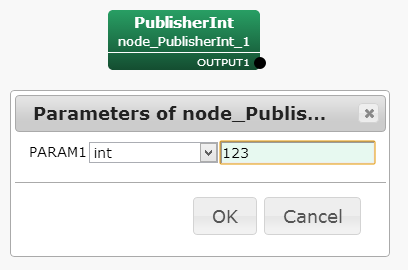Figure 13.2: SubscriberInt node input1ID= addInput("INPUT1"); This code registers the ID INPUT1 set in the GUI as input1ID defined in the cc file and allocates the GUI port to input data. ObjectRef inputtmp = getInput(input1ID, count); input1 = dereference_cast<int> (inputtmp); Data are received from the input port corresponding to its ID as an original type of HARK and are cast into int type. Like ObjectRef (Int type, Float type, Bool type, String type, etc...), an original HARK variable type is passed in the output port, with recasting performed in the receiving node in the above process. The following shows examples for other types. • int type ObjectRef inputtmp = getInput(input1ID, count); input1 = dereference_cast<int> (inputtmp); • float type ObjectRef inputtmp = getInput(input1ID, count); input1 = dereference_cast<float> (inputtmp); • bool type ObjectRef inputtmp = getInput(input1ID, count); input1 = dereference_cast<bool> (inputtmp); • string type ObjectRef inputtmp = getInput(input1ID, count); const String &input1 = object_cast<String> (inputtmp); (input1 is string type here) • Vector type RCPtr<Vector<float> > input1 = getInput(input1ID, count); ((*input1)[i] at the time of use. Same for Vector<int>,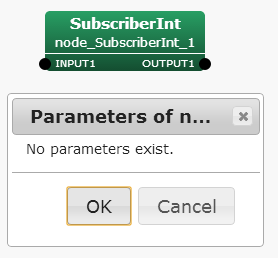• Matrix type RCPtr<Matrix<float> > input1 = getInput(input1ID, count); ((*input1)(i,j)at the time of use. Same for Matrix<int>.) The output port setting is same as for PublisherInt.cc. When finishing its creation, install it by source compilation using the same procedure as that described in the preceding chapter. Start HARK-Designer and confirm that SubscriberInt.cc has been installed properly. Create network files with PublisherInt.cc and SubscriberInt.cc Now, we will show how to create a network file (N file) in HARK using PublisherInt.cc and SubscriberInt.cc. The network file is show below; if you understand this figure, you do not need to read the rest of this chapter, so proceed to the next chapter.(a) MAIN(subnet) Sheet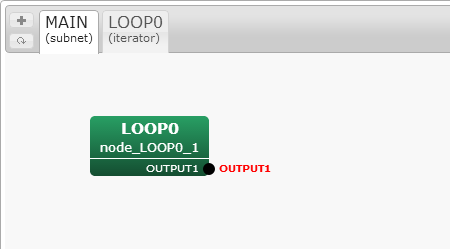(b) LOOP0(iterator) Sheet Figure 13.3: PublisherInt + SubscriberInt network file First, start HARK-Designer. For a new file, only the MAIN sheet will appear when starting-up. This is the main process in the program flow. Plus Button(Add a new sheet) > Type Iterator Add a loop processing sheet. Pick a proper sheet name (LOOP0 here). First, in the MAIN sheet side, Node list > Dynamic > LOOP0 so that LOOP0 can be executed from the MAIN. Move to the LOOP0 sheet and determine the sampling period of repetition processing (both event-based and time-based loops are possible). Here, we perform time-based repeat calculations with Sleep. Node list > Flow > Sleep (Put the Sleep node in the sheet) Double-click Sleep > Set parameter \verb|SECONDS| properly (e.g. 1.0) > OK Right-Click the output-terminal of Sleep and select "Set as condition" Confirm that the output-terminal of Sleep has become CONDITION. This is a trigger of loop processing, indicating that a new loop begins when the processing of Sleep is completed. The main processing is described next. Put two nodes in the LOOP0 sheet. Node list > HARK_TEST > PublisherInt Node list > HARK_TEST > SubscriberInt Double-click PublishInt > Set PARAM1 of PublisherInt (e.g. 123) > OK Connect the output-terminal of PublisherInt to the input of SubscriberInt Right-click the output-terminal of SubscriberInt and select "Set as output" Confirm that the output-terminal of SubscriberInt has become OUTPUT1. This is the output of loop processing. One output is created in the LOOP0 block of the MAIN sheet by adding this OUTPUT1 to the output. Go back to the MAIN sheet, and set it as follows. Right-click the output-terminal of LOOP0 and select "Set as output" Then OUTPUT1 will become the output from the MAIN sheet, enabling LOOP0 to operate. Save the file using an arbitrary name Press "Execute" The following output will appear in the console. Published : [0 , 123] Subscribed : [0 , 123] Published : [1 , 123] Subscribed : [1 , 123] Published : [2 , 123] Subscribed : [2 , 123] ... The network file that can exchange integers has been completed. The above is a basic tutorial for creating nodes. Further techniques, such as the addition of inputs and outputs and processing between multiple frames, will be described below. Adding internal parameters to a node There is one property parameter called PARAM1 in PublisherInt.cc. This section describes how to change PublisherInt.cc to arrange multiple parameters. Since the use of simple int type has been described, this section describes how to read Vector type, which is more difficult as a parameter (the new parameter is named PARAM2). The goal is to read a Vector type variable as a parameter, multiply it by PARAM1 as shown below and modify the node so that the result is output from the output port. PARAM1 * PARAM2 This tutorial involves two elements: 1) addition of an internal parameter in Vector type, and 2) addition of an output port in Vector type. Therefore, the two are described in two independent chapters. This chapter describes how to add an internal parameter. Open {$PACKAGE}/hark_test/src/PublisherInt.cc. Since we are dealing with a Vector type variable, include Vector.h first.

#include <Vector.h>

Change the GUI setting of HARK-Designer. Add the following in /*Node ... END*/.

*
* @parameter_name PARAM2
* @parameter_type Vector<int>
* @parameter_value <Vector<int> 0 1 2>
* @parameter_description OUTPUT2 = PARAM1 * PARAM2
*

Here, see @parameter_value. The format of <Vector<int> 0 1 2> is strict (e.g. spaces). Next, add the following to the member variables of the class.

Vector<int> param2;

Add the following in the constructor.

param2 = object_cast<Vector<int> >(parameters.get("PARAM2"));

Then, it can be used as Vector , as shown in the following example.

for(int i = 0;i < param2.size();i++){cout << param2[i]<< " ";}

Although this section described changes only with sentences, the finalized source code is shown in the last part of the next section.

The previous section described how to read variables of Vector type as internal parameters. In this section, PARAM1 is multiplied by the Vector type variable, and PublisherInt.cc is modified so that it can output a Vector type variable. First, add the output for GUI of HARK-Designer:

*
* @output_name OUTPUT2
* @output_type Vector<int>
* @output_description OUTPUT2 = PARAM1 * PARAM2
*

Next, add a variable for an ID of the output port to the member variables of the class.

int output2ID;

Add the following in the constructor.

Set the output in the main routine of calculate.

RCPtr<Vector<int> > output2(new Vector<int>(param2.size()));
(*(outputs[output2ID].buffer))[count]= output2;

Here, since we are calculating PARAM1 * PARAM2, the number of components in the output vector is the same as that of PARAM2. Therefore, the size of output2 is the same as that of param2.size(). Substitute PARAM1 * PARAM2 for output2.

for(int i = 0;i < param2.size();i++){
(*output2)[i]= param1 * param2[i];
}

The results of PARAM1 * PARAM2 are output from OUTPUT2. The finalized edition of PublisherInt.cc is shown below.

#include <iostream>
#include <BufferedNode.h>
#include <Buffer.h>
#include <Vector.h>

using namespace std;
using namespace HARK;

class PublisherInt;

DECLARE_NODE(PublisherInt);
/*Node
*
* @name PublisherInt
* @category HARK_TEST
* @description This block outputs the same integer as PARAM1.
*
* @output_name OUTPUT1
* @output_type int
* @output_description This output the same integer as PARAM1.
*
* @output_name OUTPUT2
* @output_type Vector<int>
* @output_description OUTPUT2 = PARAM1 * PARAM2
*
* @parameter_name PARAM1
* @parameter_type int
* @parameter_value 123
* @parameter_description Setting for OUTPUT1
*
* @parameter_name PARAM2
* @parameter_type Vector<int>
* @parameter_value <Vector<int> 0 1 2>
* @parameter_description OUTPUT2 = PARAM1 * PARAM2
*
END*/

class PublisherInt : public BufferedNode {
int output1ID;
int output2ID;
int output1;
int param1;
Vector<int> param2;

public:
PublisherInt(string nodeName, ParameterSet params) : BufferedNode(nodeName, params)
{
output1 = 0;
param1 = dereference_cast<int>(parameters.get("PARAM1"));
param2 = object_cast<Vector<int> >(parameters.get("PARAM2"));
inOrder = true;
}

void calculate(int output_id, int count, Buffer &out)
{
// Main loop routine starts here.

output1 = param1;
cout << "Published :[" << count << " , " << output1 << "]" << endl;
(*(outputs[output1ID].buffer))[count]= ObjectRef(Int::alloc(output1));

RCPtr<Vector<int> > output2(new Vector<int>(param2.size()));
(*(outputs[output2ID].buffer))[count]= output2;
cout << "Vector Published :[";
for(int i = 0;i < param2.size();i++){
(*output2)[i]= param1 * param2[i];
cout << (*output2)[i]<< " ";
}
cout << "]" << endl;

// Main loop routine ends here.
}
};

We have described a method of outputting a variable of Vector type to the new PublisherInt.cc. This chapter describes how to create a new SubscriberInt.cc that receives a Vector type variable as an input. Open {\$PACKAGE}/hark_test/src/SubscriberInt.cc using an appropriate editor. Since a Vector type variable is treated, include Vector.h first.

#include <Vector.h>

Change the GUI setting of HARK-Designer. Add the following in /*Node ... END*/.

*
* @input_name INPUT2
* @input_type Vector<int>
* @input_description input for a Vector
*

Add a member variable of the class.

int input2ID;

Add the following to the constructor.

RCPtr<Vector<int> > input2 = getInput(input2ID, count);

Then, input2 can be used in the main routine:

for(int i = 0;i < (*input2).size();i++){
cout << (*input2)[i]<< " ";
}

The finalized edition of SubscriberInt.cc is shown below.

#include <iostream>
#include <BufferedNode.h>
#include <Buffer.h>
#include <Vector.h>

using namespace std;
using namespace HARK;

class SubscriberTutorial;

DECLARE_NODE(SubscriberTutorial);
/*Node
*
* @name SubscriberTutorial
* @category HARKD:Tutorial
* @description This block inputs an integer and outputs the same number with print.
*
* @input_name INPUT1
* @input_type int
* @input_description input for an integer
*
* @input_name INPUT2
* @input_type Vector<int>
* @input_description input for a Vector
*
* @output_name OUTPUT1
* @output_type int
* @output_description Same as input
*
END*/

class SubscriberTutorial : public BufferedNode {
int input1ID;
int input2ID;
int output1ID;
int input1;

public:
SubscriberTutorial(string nodeName, ParameterSet params) : BufferedNode(nodeName, params)
{
input1 = 0;inOrder = true;
}

void calculate(int output_id, int count, Buffer &out)
{
// Main loop routine starts here.

ObjectRef inputtmp = getInput(input1ID, count);
input1 = dereference_cast<int> (inputtmp);
cout << "Subscribed :[" << count << " , " << input1 << "]" << endl;

(*(outputs[output1ID].buffer))[count] = ObjectRef(Int::alloc(input1));
RCPtr<Vector<int> > input2 = getInput(input2ID, count);

for(int i = 0;i < (*input2).size();i++){
cout << (*input2)[i]<< " ";
}
cout << "]" << endl;

// Main loop routine ends here.
}
};

Create an N file with new PublisherInt.cc and SubscriberInt.cc

The fundamental procedure is same as that for N file creation described in the previous section. First, confirm if compilation and installation were performed properly. If successful, start HARK-Designer. Open the N file created in the previous section. Confirm that: 1) OUTPUT2 has been added to the output ports of the PublisherInt node, and 2) INPUT2 has been added to the input ports of the SubsctiberInt node. (If these cannot be confirmed, installation was not successful, so trouble-shoot the problem as described in the previous section.) After connecting the two, utilize the following setting:

Double-click PublishInt > Set type of PARAM2 to "object" > OK

Save it and press "Execute".

Published :
[0 , 123]
Vector Published :
[0 123 246 ]
Subscribed :
[0 , 123]
[0 123 246 ]
Published :
[1 , 123]
Vector Published :
[0 123 246 ]
Subscribed :
[1 , 123]
[0 123 246 ]
Published :
[2 , 123]
Vector Published :
[0 123 246 ]
Subscribed :
[2 , 123]
[0 123 246 ]
...

The above is displayed in the console. Confirm that Vector is exchanged correctly. The following is the capture of the node created.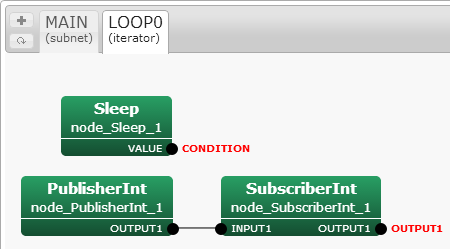Figure 13.4: SubscriberInt + PublisherInt network file

Processing over multiple frames (lookBack and lookAhead options)

The above sections have described calculations within a frame, such as multiplying a vector by a constant. However, calculations over multiple frames (e.g. means) or differentiation requires processing over multiple frames. For example, to create a block to obtain the total of input values from two frames before to two frames after the current frame (total of five frames):

 \begin{equation} OUTPUT1(t) = INPUT1(t-2) + INPUT1(t-1) + INPUT1(t) + INPUT1(t+1) + INPUT1(t+2)| \end{equation} (1)

Since getInput is maintained for each ID and count value of the input port, it can be calculated as:

total = 0.0;
for(int i = -2;i <= 2;i++){
ObjectRef inputtmp = getInput(input1ID, count + i);
input1 = dereference_cast<int> (inputtmp);
total += input1;
}

Here, the argument of getInput is expressed as "count + i", allowing processing from two frames before to two frames after the current frame. Errors during execution are due, first, to the count value having to be -2 and -1 in the first and second frames, respectively, resulting in a negative count value. This problem can be resolved simply by adding the following.

if(count >= 2)

The second reason is that in HARK  , unless particularly requested, only one frame before and one frame after the current frame are maintained during the process. Therefore, trying to see more than two frames away causes an error of undefined frames. This can be solved by describing the following in the constructor, enabling the buffer to maintain information for the desired number of frames.

inputsCache[input1ID].lookBack = 2;

The above is a command to take buffers from the two frames before and after the current frame. Set them adequately for your own purposes. This allows calculations for multiple frames. The following is the “AccumulationInt” node that takes an int type input and acquires a total number of frames, from SUM_BACKWARD frames before and SUM_FORWARD frames after the current frame. Since lookAhead and lookBack can be changed, errors will occur unless a sufficient number of frames are included in the buffer. An example of a network file is shown below.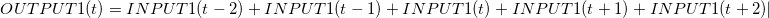(a) MAIN(subnet) Sheet(b) LOOP0(iterator) Sheet
Figure 13.5: AccumulationInt network file
#include <iostream>
#include <BufferedNode.h>
#include <Buffer.h>

using namespace std;
using namespace HARK;

class AccumulationInt;

DECLARE_NODE(AccumulationInt);
/*Node
*
* @name AccumulationInt
* @category HARKD:Tutorial
* @description This block takes a summation over several frames of the input.
*
* @input_name INPUT1
* @input_type int
* @input_description input for an integer
*
* @output_name OUTPUT1
* @output_type int
* @output_description total
*
* @parameter_name SUM_FORWARD
* @parameter_type int
* @parameter_value 5
* @parameter_description Forward buffer for summation
*
* @parameter_name SUM_BACKWARD
* @parameter_type int
* @parameter_value -5
* @parameter_description Backward buffer for summation
*
* @parameter_name LOOK_FORWARD
* @parameter_type int
* @parameter_value 0
* @parameter_description Forward buffer for summation
*
* @parameter_name LOOK_BACKWARD
* @parameter_type int
* @parameter_value 0
* @parameter_description Backward buffer for summation
*
* @parameter_name IN_ORDER
* @parameter_type bool
* @parameter_value true
* @parameter_description inOrder setting
*
END*/

class AccumulationInt : public BufferedNode {
int input1ID;
int output1ID;
int input1;
int sum_forward;
int sum_backward;
int look_forward;
int look_backward;
int total;
bool in_order;

public:
AccumulationInt(string nodeName, ParameterSet params) : BufferedNode(nodeName, params)
{
input1 = 0;
sum_forward = dereference_cast<int>(parameters.get("SUM_FORWARD"));
sum_backward = dereference_cast<int>(parameters.get("SUM_BACKWARD"));
look_forward = dereference_cast<int>(parameters.get("LOOK_FORWARD"));
look_backward = dereference_cast<int>(parameters.get("LOOK_BACKWARD"));
inputsCache[input1ID].lookBack = look_backward;
in_order = dereference_cast<bool> (parameters.get("IN_ORDER"));
inOrder = in_order;
}

void calculate(int output_id, int count, Buffer &out)
{
total = 0;
if(count + sum_backward >= 0){
for(int i = sum_backward;i <= sum_forward;i++){
ObjectRef inputtmp = getInput(input1ID, count + i);
input1 = dereference_cast<int> (inputtmp);
total += input1;
}
}
cout << "AccumulationInt :[" << count << " , " << input1 << " , " << total << "]" << endl;
(*(outputs[output1ID].buffer))[count]= ObjectRef(Int::alloc(total));
}
};

Processing that does not calculate every frame (inOrder option)

In contrast to the previous section, this chapter describes processing performed once in several frames. For example, consider the following source.

if(count % 3 == 0){
ObjectRef inputtmp = getInput(input1ID, count);
input1 = dereference_cast<int> (inputtmp);
}

In this case, getInput is not read for every count, but once in three frames. In HARK  , the former node is processed in accordance with the request from the getInput. That is, with a source like the above, the node request inputs once in three frames. Therefore, its former node is calculated once in three frames. The following is an example. First, as a preparation, create the following SubscriberIntWithPeriod node.

#include <iostream>
#include <BufferedNode.h>
#include <Buffer.h>

using namespace std;
using namespace HARK;

class SubscriberIntWithPeriod;

DECLARE_NODE(SubscriberIntWithPeriod);
/*Node
*
* @name SubscriberIntWithPeriod
* @category HARKD:Tutorial
* @description This block inputs an integer and outputs the same number with print with specific period.
*
* @input_name INPUT1
* @input_type int
* @input_description input for an integer
*
* @output_name OUTPUT1
* @output_type int
* @output_description Same as input
*
* @parameter_name PERIOD
* @parameter_type int
* @parameter_value 1
* @parameter_description Period of INPUT1 subscription
*
* @parameter_name IN_ORDER
* @parameter_type bool
* @parameter_value true
* @parameter_description inOrder setting
*
END*/

class SubscriberIntWithPeriod : public BufferedNode {
int input1ID;
int output1ID;
int input1;
int period;
bool in_order;

public:
SubscriberIntWithPeriod(string nodeName, ParameterSet params) : BufferedNode(nodeName, params){
input1 = 0;
period = dereference_cast<int>(parameters.get("PERIOD"));
in_order =dereference_cast<bool> (parameters.get("IN_ORDER"));
inOrder = in_order;
}

void calculate(int output_id, int count, Buffer &out){
// Main loop routine starts here.
if(count % period == 0){
ObjectRef inputtmp = getInput(input1ID, count);
input1 = dereference_cast<int> (inputtmp);
}
cout << "Subscribed :[" << count << " , " << input1 << "]" << endl;
(*(outputs[output1ID].buffer))[count]= ObjectRef(Int::alloc(input1));
// Main loop routine ends here.
}
};

In the SubscriberIntWithPeriod node, getInput is performed over the period set in PERIOD, and its value is displayed. Next, create the CountOutput node to output the current count value without changing it.

#include <iostream>
#include <BufferedNode.h>
#include <Buffer.h>

using namespace std;
using namespace HARK;

class CountOutput;

DECLARE_NODE(CountOutput);
/*Node
*
* @name CountOutput
* @category HARKD:Tutorial
* @description This block outputs the count number
*
* @output_name OUTPUT1
* @output_type int
* @output_description This output the same integer as PARAM1.
*
* @parameter_name IN_ORDER
* @parameter_type bool
* @parameter_value true
* @parameter_description inOrder setting
*
END*/

class CountOutput : public BufferedNode {
int output1ID;
bool in_order;

public:
CountOutput(string nodeName, ParameterSet params): BufferedNode(nodeName, params)
{
in_order = dereference_cast<bool> (parameters.get("IN_ORDER"));
inOrder = in_order;
}

void calculate(int output_id, int count, Buffer &out){
cout << "CountOut :[" << count << "]" << endl;
(*(outputs[output1ID].buffer))[count] = ObjectRef(Int::alloc(count));
}
};

When the two nodes are completed, build a network file of HARK-Designer.(a) MAIN(subnet) Sheet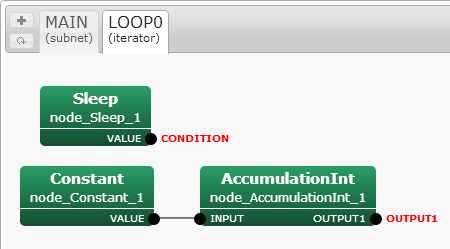(b) LOOP0(iterator) Sheet
Figure 13.6: SubscriberIntWithPeriod network file

First, set IN_ORDER of both CountOutput and SubscriberIntWithPeriod to "false" (These are default settings). Set PERIOD of SubscriberIntWithPeriod as 3, and execute the network file. The output of the console will be:

CountOut :

Subscribed :
[0 , 0]
Subscribed :
[1 , 0]
Subscribed :
[2 , 0]
CountOut :

Subscribed :
[3 , 3]
Subscribed :
[4 , 3]
Subscribed :
[5 , 3]
CountOut :

Subscribed :
[6 , 6]
Subscribed :
[7 , 6]
Subscribed :
[8 , 6]
CountOut :

Subscribed :
[9 , 9]
...

Thus, since the latter SubscriberIntWithPeriod request inputs once in three frames, CountOut will be processed only once every three frames. If processing such as differentiation or count calculation is performed in CountOut, the process cannot be performed correctly. (To confirm this problem, implement a counter that counts every time calculate is called by the CountOut node. This will confirm that the counter does not work for all count.) The ”inOrder” option solves this problem. Set IN_ORDER to true in the abovementioned network file. When executed, the following result is obtained:

CountOut :

Subscribed :
[0 , 0]
Subscribed :
[1 , 0]
Subscribed :
[2 , 0]
CountOut :

CountOut :

CountOut :

Subscribed :
[3 , 3]
Subscribed :
[4 , 3]
Subscribed :
[5 , 3]
CountOut :

CountOut :

CountOut :

Subscribed :
[6 , 6]
Subscribed :
[7 , 6]
Subscribed :
[8 , 6]
CountOut :

CountOut :

CountOut :

Subscribed :
[9 , 9]
...

Thus, loop processing is properly executed for CountOut three times, in agreement with the requirement that it be performed once every three frames. To properly calculate the number of count times for all nodes, regardless of requirements, enter the following into the constructor:

inOrder = true;

Creating a node that takes a dynamic number of inputs (translateInput)

This section describes how to create a node that takes a dynamic number of inputs. The following example takes three int type inputs and calculates their total.

#include <iostream>
#include <BufferedNode.h>
#include <Buffer.h>

using namespace std;
using namespace HARK;

class SummationInt;

DECLARE_NODE(SummationInt);
/*Node
*
* @name SummationInt
* @category HARKD:Tutorial
* @description This block outputs INPUT1 + INPUT2 + INTPUT3
*
* @input_name INPUT1
* @input_type int
* @input_description input for an integer
*
* @input_name INPUT2
* @input_type int
* @input_description input for an integer
*
* @input_name INPUT3
* @input_type int
* @input_description input for an integer
*
* @output_name OUTPUT1
* @output_type int
* @output_description Same as input
*
END*/

class SummationInt : public BufferedNode {
int input1ID;
int input2ID;
int input3ID;
int output1ID;
int input1;
int input2;
int input3;
int total;

public:
SummationInt(string nodeName, ParameterSet params) : BufferedNode(nodeName, params){
inOrder = true;
}

void calculate(int output_id, int count, Buffer &out){
// Main loop routine starts here.

input1 = 0;
input2 = 0;
input3 = 0;
ObjectRef inputtmp1 = getInput(input1ID, count);
input1 = dereference_cast<int> (inputtmp1);
ObjectRef inputtmp2 = getInput(input2ID, count);
input2 = dereference_cast<int> (inputtmp2);
ObjectRef inputtmp3 = getInput(input3ID, count);
input3 = dereference_cast<int> (inputtmp3);
total = input1 + input2 + input3;
cout << "SummationInt :[" << count << " , " << total << "]" << endl;
(*(outputs[output1ID].buffer))[count]= ObjectRef(Int::alloc(total));

// Main loop routine ends here.
}
};

When the node is created, create a network file as follows.(a) MAIN(subnet) Sheet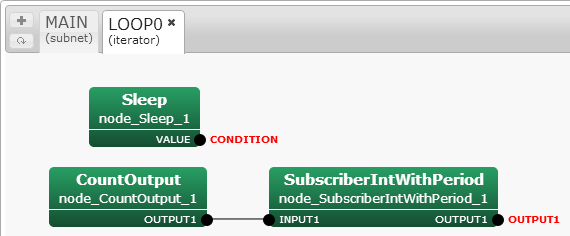(b) LOOP0(iterator) Sheet
Figure 13.7: SummationInt network file

In this case, 1+1+1, so 3 is the result. Currently, this node has to take three inputs. Now, suppose that you wish to sum two variables from this node, not all three. It thus appears that these calculations can be performed by opening one input port and giving integers to two ports, as shown in the following figure. However, when executing, an error occurred because the node requires all input ports to be connected.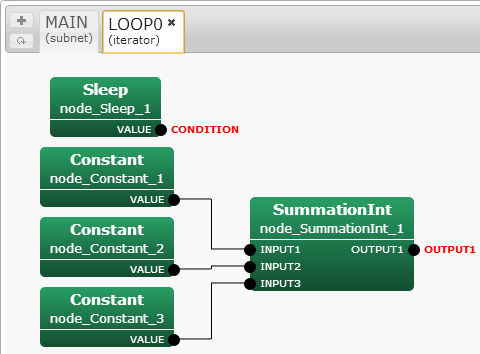Figure 13.8: SummationInt network file (input port imperfection)

In HARK  , except in special cases, all information about the input ports read in getInput must be received, even if all the data are not used for processing inside the node. To realize the dynamic number of inputs, you can use a method called translateInput, which is in the member function of the Node class, which is a parent class of BufferedNode. To create a node in which the number of input ports varies with the same example.

#include <iostream>
#include <BufferedNode.h>
#include <Buffer.h>

using namespace std;
using namespace HARK;

class SummationInt;

DECLARE_NODE(SummationInt);
/*Node
*
* @name SummationInt
* @category HARKD:Tutorial
* @description This block outputs INPUT1 + INPUT2 + INTPUT3
*
* @input_name INPUT1
* @input_type int
* @input_description input for an integer
*
* @input_name INPUT2
* @input_type int
* @input_description input for an integer
*
* @input_name INPUT3
* @input_type int
* @input_description input for an integer
*
* @output_name OUTPUT1
* @output_type int
* @output_description Same as input
*
END*/

class SummationInt : public BufferedNode {
int input1ID;
int input2ID;
int input3ID;
int output1ID;
int input1;
int input2;
int input3;
int total;

public:
SummationInt(string nodeName, ParameterSet params)
: BufferedNode(nodeName, params), input1ID(-1), input2ID(-1), input3ID(-1)
{
inOrder = true;
}

virtual int translateInput(const string &inputName){
if (inputName == "INPUT1"){return input1ID = addInput(inputName);}
else if (inputName == "INPUT2"){return input2ID = addInput(inputName);}
else if (inputName == "INPUT3"){return input3ID = addInput(inputName);}
else {throw new NodeException(this, inputName+ " is not supported.", __FILE__, __LINE__);}
}

void calculate(int output_id, int count, Buffer &out){
// Main loop routine starts here.
input1 = 0;
input2 = 0;
input3 = 0;
if (input1ID != -1){
ObjectRef inputtmp1 = getInput(input1ID, count);
input1 = dereference_cast<int> (inputtmp1);
}
if (input2ID != -1){
ObjectRef inputtmp2 = getInput(input2ID, count);
input2 = dereference_cast<int> (inputtmp2);
}
if (input3ID != -1){
ObjectRef inputtmp3 = getInput(input3ID, count);
input3 = dereference_cast<int> (inputtmp3);
}
total = input1 + input2 + input3;
cout << "SummationInt :[" << count << " , " << total << "]" << endl;
(*(outputs[output1ID].buffer))[count] = ObjectRef(Int::alloc(total));
// Main loop routine ends here.
}
};

The changes are as follows.

• The input port ID is initialized with -1 in the constructor.
Thus, the ID will be kept at -1 unless the input port is connected.

This function detects which ports are connected. If an input port is connected, its ID will be acquired.

• Processing is performed in calculate for the input ports whose value is other than -1.
This allows processing of examples in which the input is either opened or unopened.

Compiling this source and using it in HARK  will show that the calculation has been performed properly, without depending on the number of inputs.